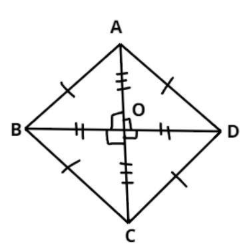Courses
Courses for Kids
Free study material
Free LIVE classes
MoreLIVE
Join Vedantu’s FREE Mastercalss

# In the rhombus ABCD, show that $4{\left( {AB} \right)^2} = {\left( {AC} \right)^2} + {\left( {BD} \right)^2}$Verified
361.8k+ views
Hint – Take any one of the four triangles and apply PGT in it after using the few properties of the Rhombus, that is diagonals are perpendicular bisectors of each other and all sides are equal.
ABCD rhombus is shown above.
As we know in rhombus diagonals bisect each other and are perpendicular to each other.
$\Rightarrow OB = OD,{\text{ & }}OA = OC..................\left( 1 \right) \\ \angle AOB = \angle AOD = \angle BOC = \angle DOC = {90^0} \\$
And also we know that all the sides of the rhombus are equal.
$\Rightarrow AB = BC = CD = DA$………………. (2)
So in triangle AOB apply Pythagoras Theorem
${\left( {{\text{Hypotenuse}}} \right)^2} = {\left( {{\text{Perpendicular}}} \right)^2} + {\left( {{\text{Base}}} \right)^2}$
$\Rightarrow {\left( {AB} \right)^2} = {\left( {OA} \right)^2} + {\left( {OB} \right)^2}$…………. (3)
Now from figure $AC = OA + OC,{\text{ }}BD = BO + OD$
From equation (2)
$AC = OA + OA,{\text{ }}BD = OB + OB \\ \Rightarrow OA = \dfrac{{AC}}{2},{\text{ }}OB = \dfrac{{BD}}{2} \\$
Now from equation (3)
$\Rightarrow {\left( {AB} \right)^2} = {\left( {\dfrac{{AC}}{2}} \right)^2} + {\left( {\dfrac{{BD}}{2}} \right)^2} \\ \Rightarrow {\left( {AB} \right)^2} = \dfrac{{{{\left( {AC} \right)}^2}}}{4} + \dfrac{{{{\left( {BD} \right)}^2}}}{4} \\ \Rightarrow 4{\left( {AB} \right)^2} = {\left( {AC} \right)^2} + {\left( {BD} \right)^2} \\$
Hence Proved.

Note – In such types of questions the key concept we have to remember is that always recall the condition of Rhombus which is stated above in equation (1) and (2), then use the property of Pythagoras theorem which is also stated above and simplify according to properties of rhombus, we will get the required result.
Last updated date: 21st Sep 2023
Total views: 361.8k
Views today: 4.61k